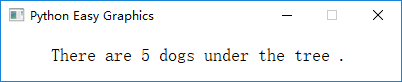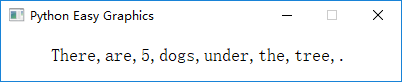# 绘制文字¶

```from easygraphics import *

def main():
init_graph(400, 50)
draw_text(50, 30, "There", "are", 5, "dogs", "under", "the", "tree", ".")
pause()
close_graph()

easy_run(main)
``````from easygraphics import *

def main():
init_graph(400, 50)
draw_text(50, 30, "There", "are", 5, "dogs", "under", "the", "tree", ".", sep=",")
pause()
close_graph()

easy_run(main)
```## 文本绘制的位置¶

```from easygraphics import *

def main():
init_graph(500, 200)
draw_text(50, 0, "Hello world!")
pause()
close_graph()

easy_run(main)
```

## 在指定的矩形区域中绘制文字¶

```from easygraphics import *

def main():
init_graph(400, 300)
draw_rect(50, 50, 350, 250)  # draw a border to better see the result
draw_rect_text(50, 50, 300, 200, "There are so many beautiful flowers in the garden!",
flags=TextFlags.TEXT_WORD_WRAP | TextFlags.ALIGN_CENTER)
pause()
close_graph()

easy_run(main)
```